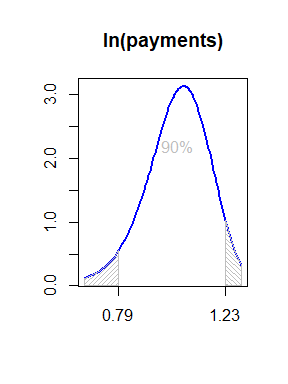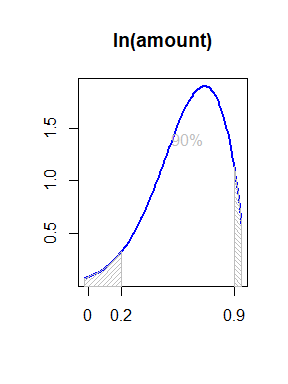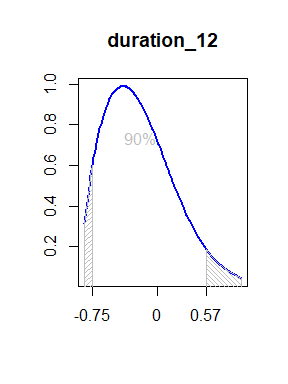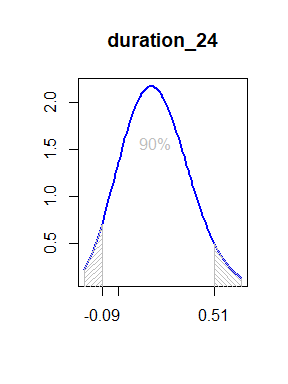# Binary Regression (loan default)

library(ldt)

## Introduction

The search.bin() function is one of the three main functions in the ldt package. This vignette explains a basic usage of this function using Berka and Sochorova (1993) dataset. Loan default refers to the failure to repay a loan according to the terms agreed upon in the loan contract. This topic has been extensively studied in the literature. According to Manz (2019), determinants of default can include macroeconomic events such as changes in interest and unemployment rates, bank-specific factors such as risk management strategies, and loan-specific factors such as its amount and purpose, as well as borrower creditworthiness. In this section, we will use the ldt package to conduct an experiment on this topic while making minimal theoretical assumptions.

## Data

Berka and Sochorova (1993) dataset has a loan table with 682 observations, each labeled as finished, finished with default, running, and running with default. Each loan observation has an account identification that can provide other types of information from other tables, such as the characteristics of the account of the loan and its transactions. Each account has a district identification that can provide information about the demographic characteristics of the location of its branch. The combined table has 58 features and 682 observations.

For this example, we use just the first 7 columns of data:

data <- cbind(data.berka$y, data.berka$x[,1:7])

Here are the last few observations from this subset of the data:

tail(data)
#>        label ln(amount) ln(payments) rate duration_12 duration_36 duration_60
#> [677,]     0   11.98866     8.405144    0           0           1           0
#> [678,]     0   12.77338     8.902183    0           0           0           0
#> [679,]     0   10.86880     8.383890    0           1           0           0
#> [680,]     0   11.84573     8.667680    0           0           0           0
#> [681,]     0   10.92651     7.748460    0           0           0           0
#> [682,]     0   12.39214     8.297793    0           0           0           1
#>        duration_24
#> [677,]           0
#> [678,]           0
#> [679,]           0
#> [680,]           1
#> [681,]           1
#> [682,]           0

And here are some summary statistics for each variable:

sapply(as.data.frame(data), summary)
#>            label ln(amount) ln(payments) rate duration_12 duration_36
#> Min.    0.000000   8.513185     5.717028    0   0.0000000   0.0000000
#> 1st Qu. 0.000000  11.108439     7.814803    0   0.0000000   0.0000000
#> Median  0.000000  11.669313     8.277412    0   0.0000000   0.0000000
#> Mean    0.111437  11.614518     8.157249    0   0.1920821   0.1906158
#> 3rd Qu. 0.000000  12.257972     8.667938    0   0.0000000   0.0000000
#> Max.    1.000000  13.289267     9.201300    0   1.0000000   1.0000000
#>         duration_60 duration_24
#> Min.        0.00000    0.000000
#> 1st Qu.     0.00000    0.000000
#> Median      0.00000    0.000000
#> Mean        0.21261    0.202346
#> 3rd Qu.     0.00000    0.000000
#> Max.        1.00000    1.000000

The columns of the data represent the following variables:

• label: default=1

• ln(amount): amount of money (log)

• ln(payments): monthly payments (log)

• rate: interest rate

• duration_12: dummy variable indicating a 12-month loan duration

• duration_36: dummy variable indicating a 36-month loan duration

• duration_60: dummy variable indicating a 60-month loan duration

• duration_24: dummy variable indicating a 24-month loan duration

## Modelling

The target variable is the first variable. We use the out-of-sample AUC evaluation metric to find the best predicting model.


search_res <- search.bin(data = get.data(data, endogenous = 1, weights = data.berka$w), combinations = get.combinations(sizes = c(1,2,3), numTargets = 1), metric <- get.search.metrics(typesIn = c(), typesOut = c("auc"), simFixSize = 20, trainRatio = 0.8, seed = 123), items = get.search.items(bestK = 0, inclusion = TRUE, type1 = TRUE, mixture4 = TRUE)) print(search_res) #> LDT search result: #> Method in the search process: Binary #> Expected number of models: 29, searched: 29 , failed: 7 (24.1%) #> Elapsed time: 0.01655978 minutes #> Length of results: 2 #> -------- #> Failures: #> 1. ldt::statistics->matrix singularity: 7 (24.1%) #> -------- #> Target (label): #> Evaluation (aucOut): #> Inclusion weights average: #> maximum value: 0.6738315 #> name: ln(payments) #> count: 6 #> Mixture significant [mean-1.95*std, mean, mean+1.95*std]: #> ln(amount): (3x1) 0.1843058, 0.6110107, 1.037716 #> ln(payments): (3x1) 0.7696544, 1.032932, 1.296209 #> -------- The output of the search.bin() function does not contain any estimation results, but only the information required to replicate them. The summary() function returns a similar structure but with the estimation results included. search_sum <- summary(search_res) While choosing an out-of-sample metric indicates our interest in the predictive power of the best model, for presentation purposes, the mixture4 = TRUE part is also included in the searchItems argument. Therefore, we use the results to study the coefficients uncertainty. In this regard, we select the first four regressors with the highest inclusion weights (note the inclusion = TRUE) and plot their combined weighted distributions. Note that the parameters of the generalized lambda distribution are estimated from the first four moments of the combined distribution using the s.gld.from.moments()} function in this package, with the distribution restricted to be unimodal with a continuous tail. First, we prepare data for plot:  inclusion_mat <- search_res$results[sapply(search_res$results, function(a)a$typeName == "inclusion")][]$value inclusion_mat <- inclusion_mat[!(rownames(inclusion_mat) %in% c("label", "(Intercept)")), ] sorted_inclusion_mat <- inclusion_mat[order(inclusion_mat[,1], decreasing = TRUE),] selected_vars <- rownames(sorted_inclusion_mat)[1:4] mixture_mat <- search_sum$results[sapply(search_sum$results, function(a)a$typeName == "mixture")][]\$value
moments <- lapply(selected_vars, function(v)mixture_mat[rownames(mixture_mat)==v,])
gld_parms <- lapply(moments, function(c) s.gld.from.moments(c[], c[],
c[], c[],
start = c(0.25, 0.25),
type = 4,
nelderMeadOptions = get.options.neldermead(100000,1e-6)))

Then we plot the estimated distributions:


# plots
probs <- seq(0.01,0.99,0.01)
i <- 0
for (gld in gld_parms){
i <- i + 1
x <- s.gld.quantile(probs, gld,gld,gld,gld)
y <- s.gld.density.quantile(probs, gld,gld,gld,gld)

plot(x, y, type = "l", xaxt = "n", xlab = NA, ylab = NA, col = "blue", lwd = 2,
main = selected_vars[i])
lower <- x[abs(probs - 0.05)<1e-10]
upper <- x[abs(probs - 0.95)<1e-10]
axis(1, at = c(lower, 0, upper), labels = c(round(lower, 2), 0, round(upper, 2)))
xleft <- x[x <= lower]
xright <- x[x >= upper]
yleft <- y[x <= lower]
yright <- y[x >= upper]
polygon(c(min(x), xleft, lower), c(0, yleft, 0), col = "gray", density = 30, angle = 45)
polygon(c(max(x), upper, xright), c(0, 0, yright), col = "gray", density = 30, angle = -45)
text(mean(x), mean(y), "90%", col = "gray")
}Determinants of Loan Default: Variables with the highest inclusion weights, automatically selected. Each plot presents a combined distribution of all estimated coefficients, estimated by generalized lambda distribution.

## Conclusion

This package can be a recommended tool for empirical studies that require reducing assumptions and summarizing uncertainty analysis results. This vignette is just a demonstration. There are indeed other options you can explore with the search.bin() function. For instance, you can experiment with different evaluation metrics or restrict the model set based on your specific needs. Additionally, there’s an alternative approach where you can combine modeling with Principal Component Analysis (PCA) (see estim.bin()` function). I encourage you to experiment with these options and see how they can enhance your data analysis journey.